# Indefinite Integrals

In Calculus, the two important processes are differentiation and integration. We know that differentiation is the process of finding the derivative of a function, whereas integration is the inverse process of differentiation. Here, we are going to discuss the important component of integration called “integrals” here. The integrals are generally classified into two types, namely:

• Definite Integral
• Indefinite Integral

Here, let us discuss one of the types of integral called “Indefinite Integral” with definition and properties in detail.

## Indefinite Integrals Definition

An integral which is not having any upper and lower limit is known as an indefinite integral.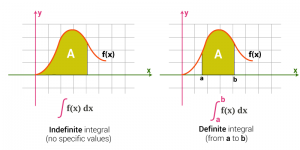F(x) is the way function f(x) is integrated and it is represented by: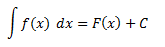Where in respect to x the integral of f(x) is on the R.H.S.

primitive or anti-derivative is termed as F(x)

Integrand is termed as f(x)

Integrating agent is termed as dx

The constant of integration is an arbitrary constant termed as C

The variable of integration is termed as x

## Properties of Indefinite Integrals

Let us now look into some properties of indefinite integrals.

Property 1:

Differentiation and integration are inverse processes of each other since: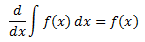and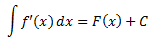Where C is any arbitrary constant. Let us now prove this statement.

Proof: Consider a function f such that its anti-derivative is given by F, i.e.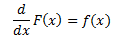Then,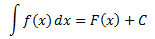On differentiating both the sides with respect to x we have,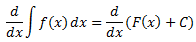Since derivative of any constant function is zero, therefore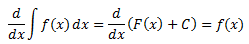The derivative of a function f in x is given as f’(x), therefore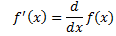Thus,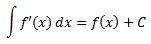Where, C is an arbitrary constant called as the constant of integration.

Property 2: Two indefinite integrals having the same derivative have the same family of integrals or curves and therefore they are equivalent.

Proof:

Consider two functions f and g in x such that: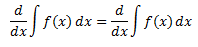Or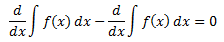On integrating both sides, we get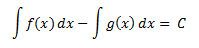where C is any real number.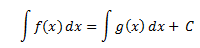From this equation, we can say that the family of the curves of [ ∫ f(x)dx + c3, c3∈ R] and  [ ∫ g(x)dx + c2, c2∈ R] are same. Therefore, we cay say that, ∫ f(x)dx = ∫ g(x)dx

Property 3:

The integral of the sum of two functions is equal to the sum of integrals of the given functions, i.e.,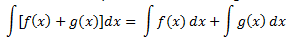Proof: By the first property of integrals we have,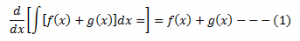Also,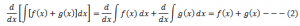Therefore from equation (1) and (2) we have,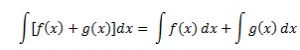Property 4: For any real value of p,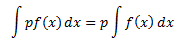Proof: From property 1 we can say that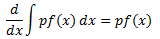Also,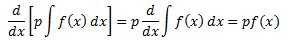From property 2 we can say that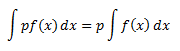Property 5:

For a finite number of functions f1, f2…. fn and the real numbers p1, p2…pn,

∫[p1f1(x) + p2f2(x)….+pnfn(x) ]dx = p1∫f1(x)dx +  p2∫f2(x)dx + ….. +  pn∫fn(x)dx

### Indefinite Integrals Problems

Go through the following integral problems given below:

Example 1:

Evaluate the given indefinite integral problem:∫6x5 -18x2 +7 dx

Solution:

Given:

∫6x5 -18x2 +7 dx

Integrate the given function, it becomes:

∫6x5 -18x2 +7 dx = 6(x6/6) – 18 (x3/3) + 7x + C

Note: Don’t forget to put the integration constant “C”

After simplification, we get the solution

Thus, ∫6x5 -18x2 +7 dx = x6-6x3+ 7x+ C

Example 2:

Evaluate f(x), given that f ‘(x) = 6x8 -20x4 + x2 + 9

Solution:

Given that, f ‘(x) = 6x8 -20x4 + x2 + 9

We know that, the inverse process of differentiation is an inetgration.

Thus, f(x) = ∫f ‘(x) dx=∫[6x8 -20x4 + x2 + 9] dx

f(x) = (2/3)x9 – 4x5 +(1/3)x3 + 9x+ C